# 一文读懂！异常检测全攻略！从统计方法到机器学习 ⛵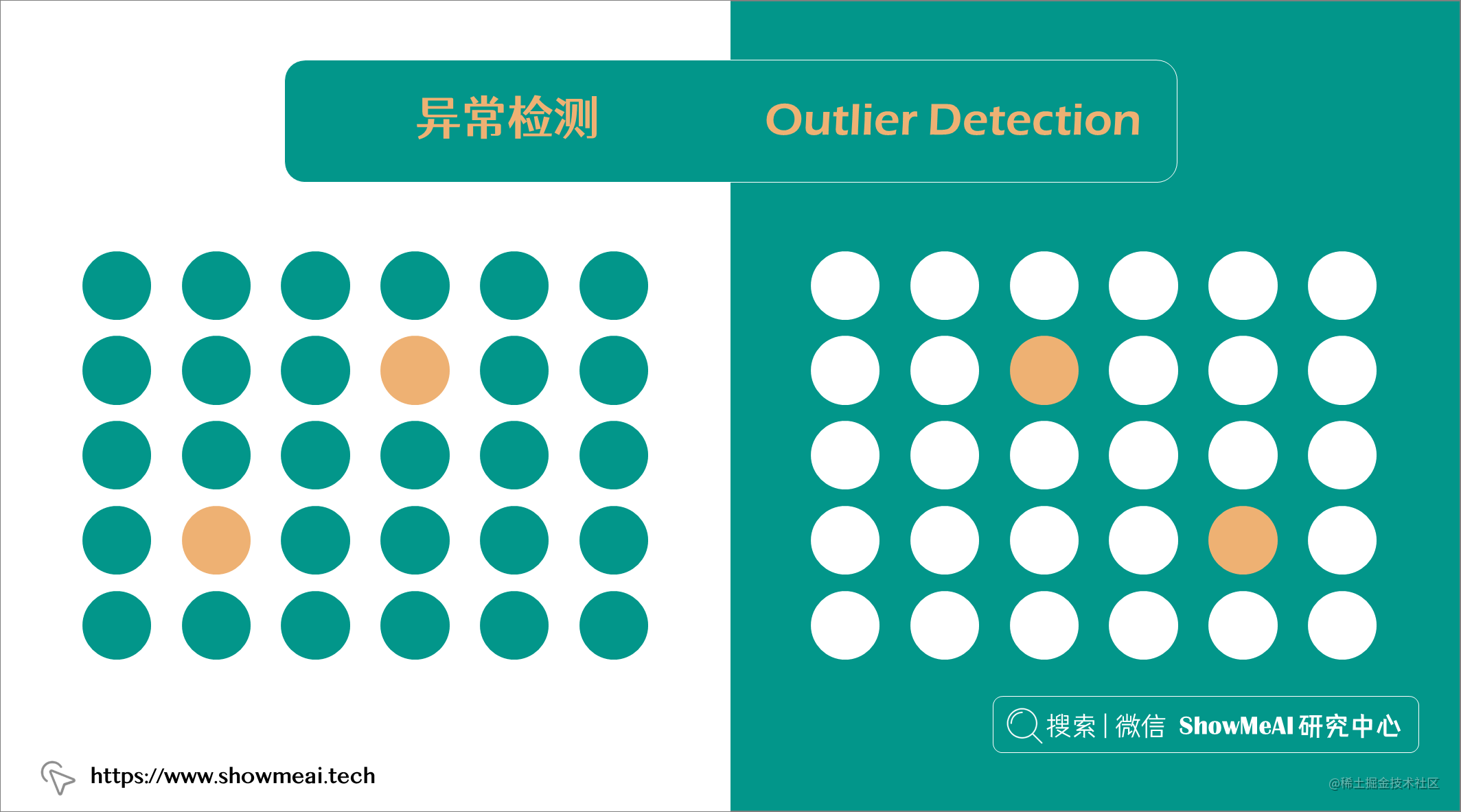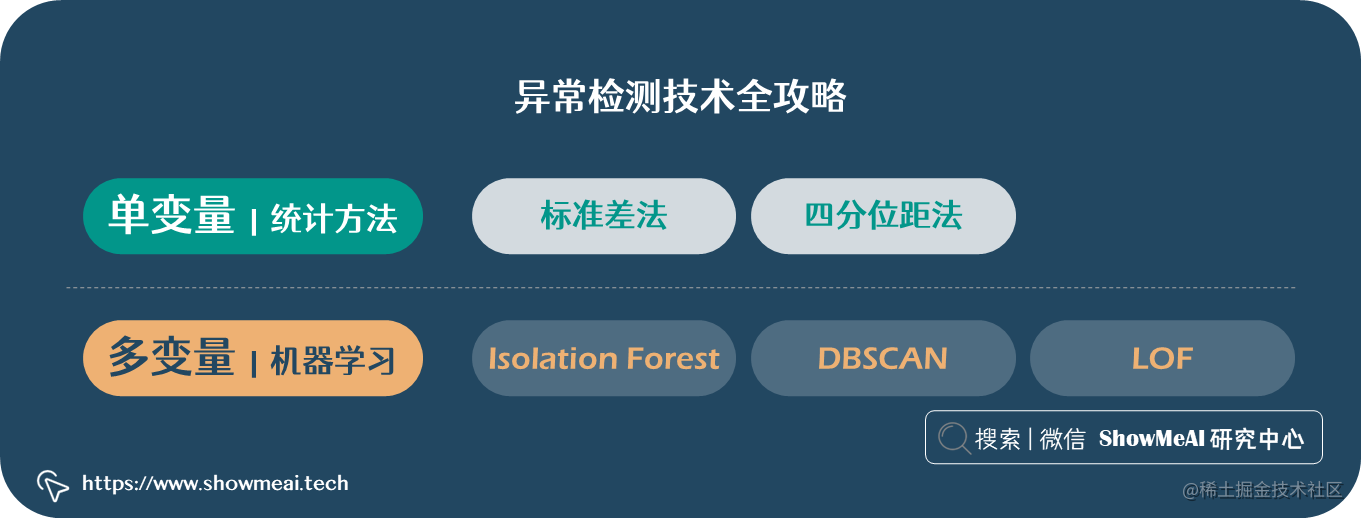# 💡 数据&场景方法概述

## 💦 数据

🏆 实战数据集下载（百度网盘）：公众号『ShowMeAI研究中心』回复『实战』，或者点击 这里 获取本文 异常检测实战全景图：从统计方法到机器学习glass数据集

ShowMeAI官方GitHubgithub.com/ShowMeAI-Hu…

``````import pandas as pd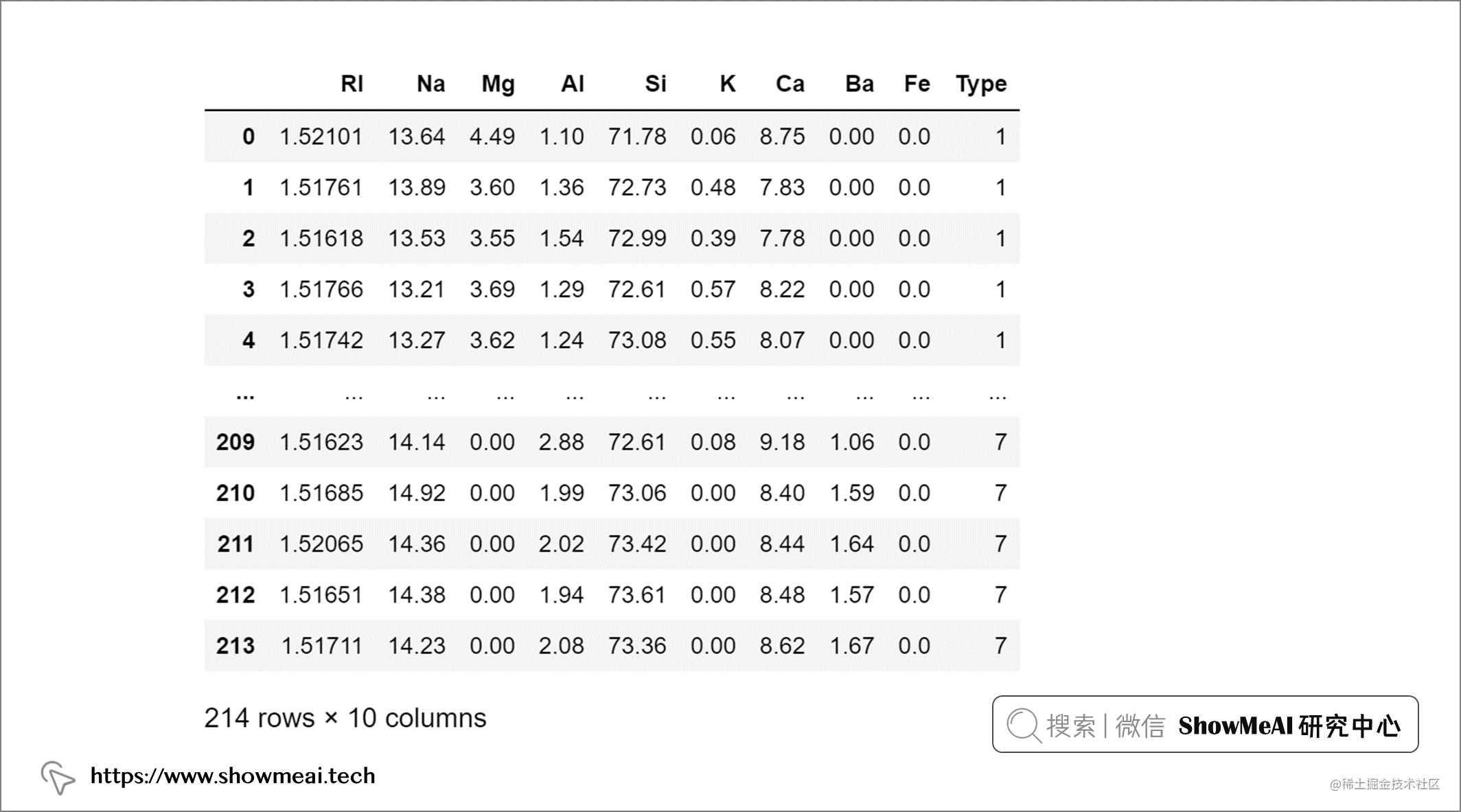## 💦 单变量和多变量异常值``````import seaborn as sns
sns.pairplot(glass, diag_kws={'color':'red'})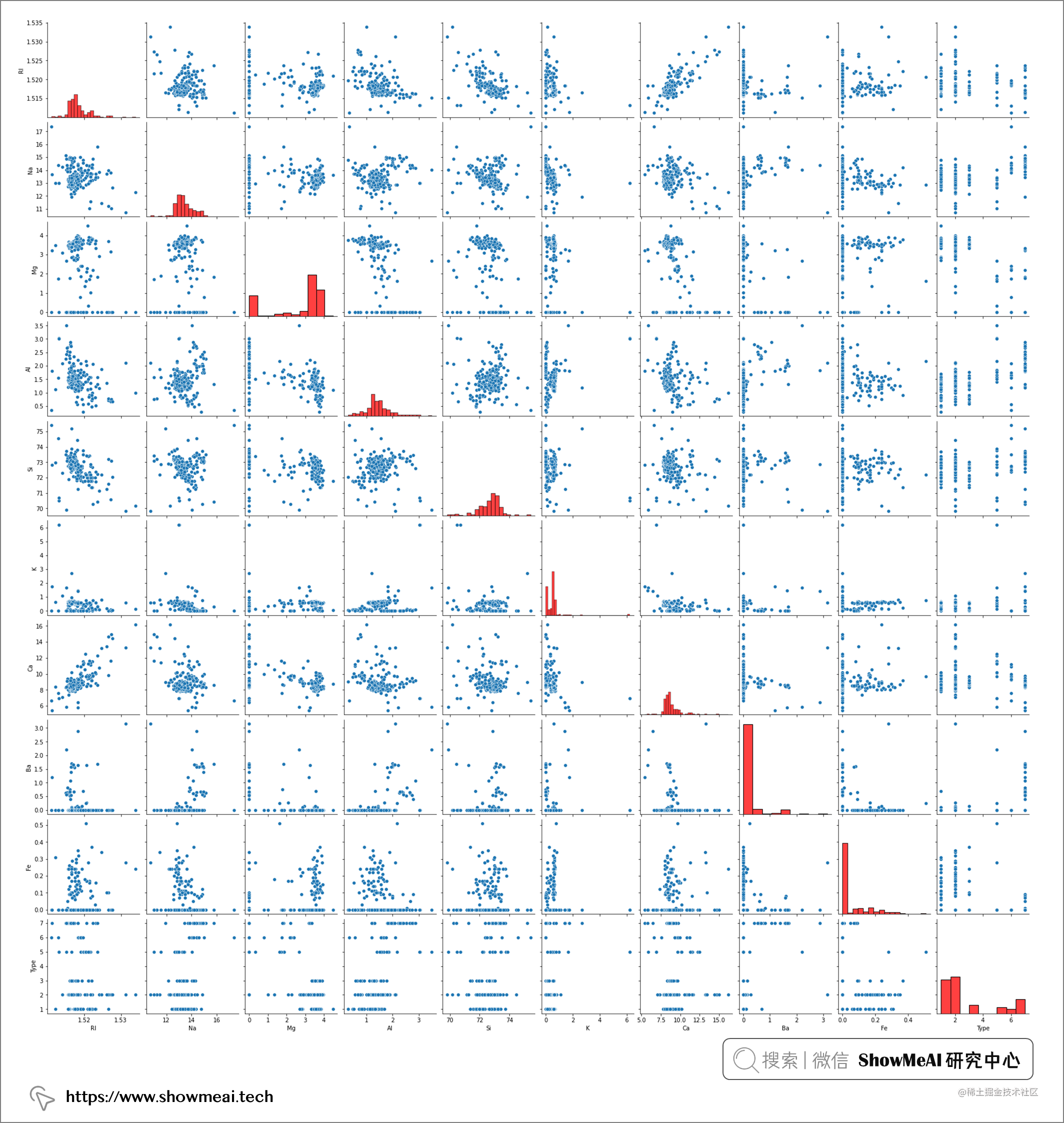pairplot 的结果包含两两数据的关联分析和每个变量的分布结果，其中对角线为单变量的分布可视化，我们发现并非所有属性字段都具有遵循正态分布。*事实上，大多数属性都偏向较低值（即 Ba、Fe）或较高值（即 Mg）**。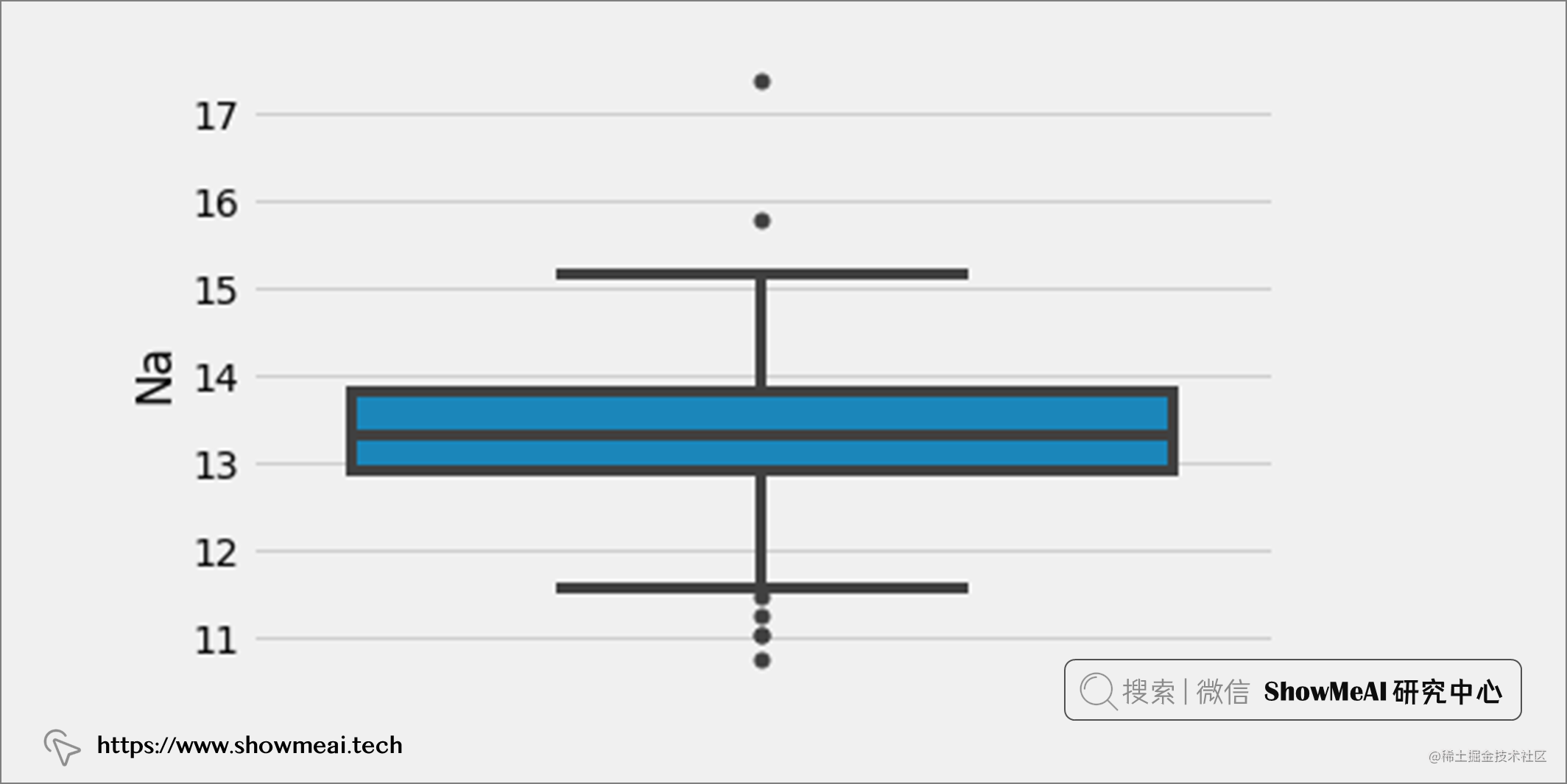``````from sklearn.decomposition import PCA
import plotly.express as px

# Dimensionality reduction to 3 dimensions
pca = PCA(n_components=3)
glass_pca = pca.fit_transform(glass.iloc[:, :-1])

# 3D scatterplot
fig = px.scatter_3d(x=glass_pca[:, 0],
y=glass_pca[:, 1],
z=glass_pca[:, 2],
color=glass.iloc[:, -1])
fig.show()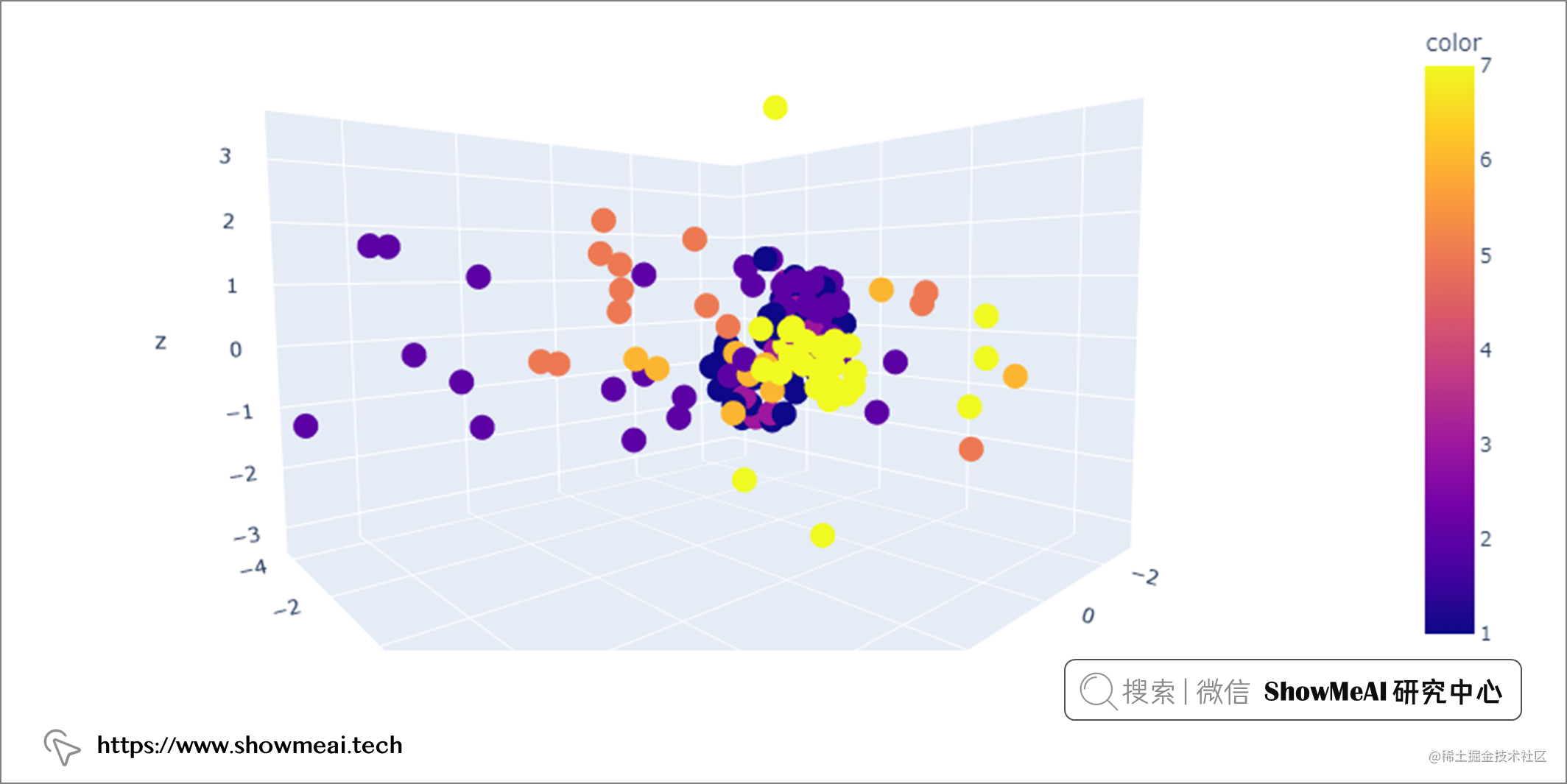# 💡 单变量异常值检测

## 💦 标准差法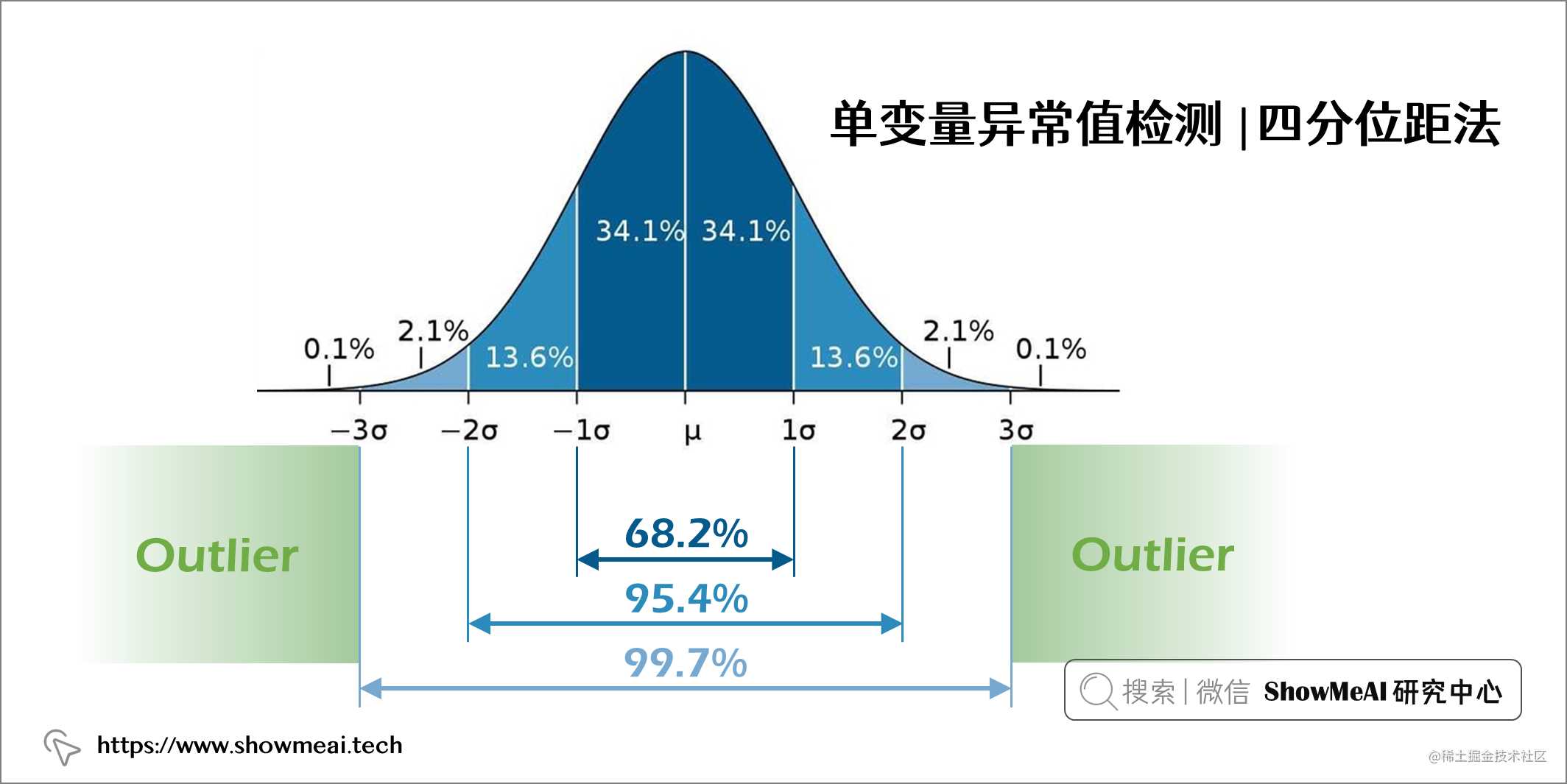``````# Find mean, standard deviation and cut off value
mean = glass["Na"].mean()
std = glass["Na"].std()
cutoff = 3 * std

# Define lower and upper boundaries
lower, upper = mean-cutoff, mean+cutoff

# Define new dataset by masking upper and lower boundaries
new_glass = glass[(glass["Na"] > lower) & (glass["Na"] < upper)]

``````Shape of original dataset: (213, 9)
Shape of dataset after removing outliers in Na column: (211, 9)

## 💦 四分位距法

• 四分位数 1 (Q1) 表示第 25 个百分位数
• 四分位数 2 (Q2) 表示第 50 个百分位数
• 四分位数 3 (Q3) 表示第 75 个百分位数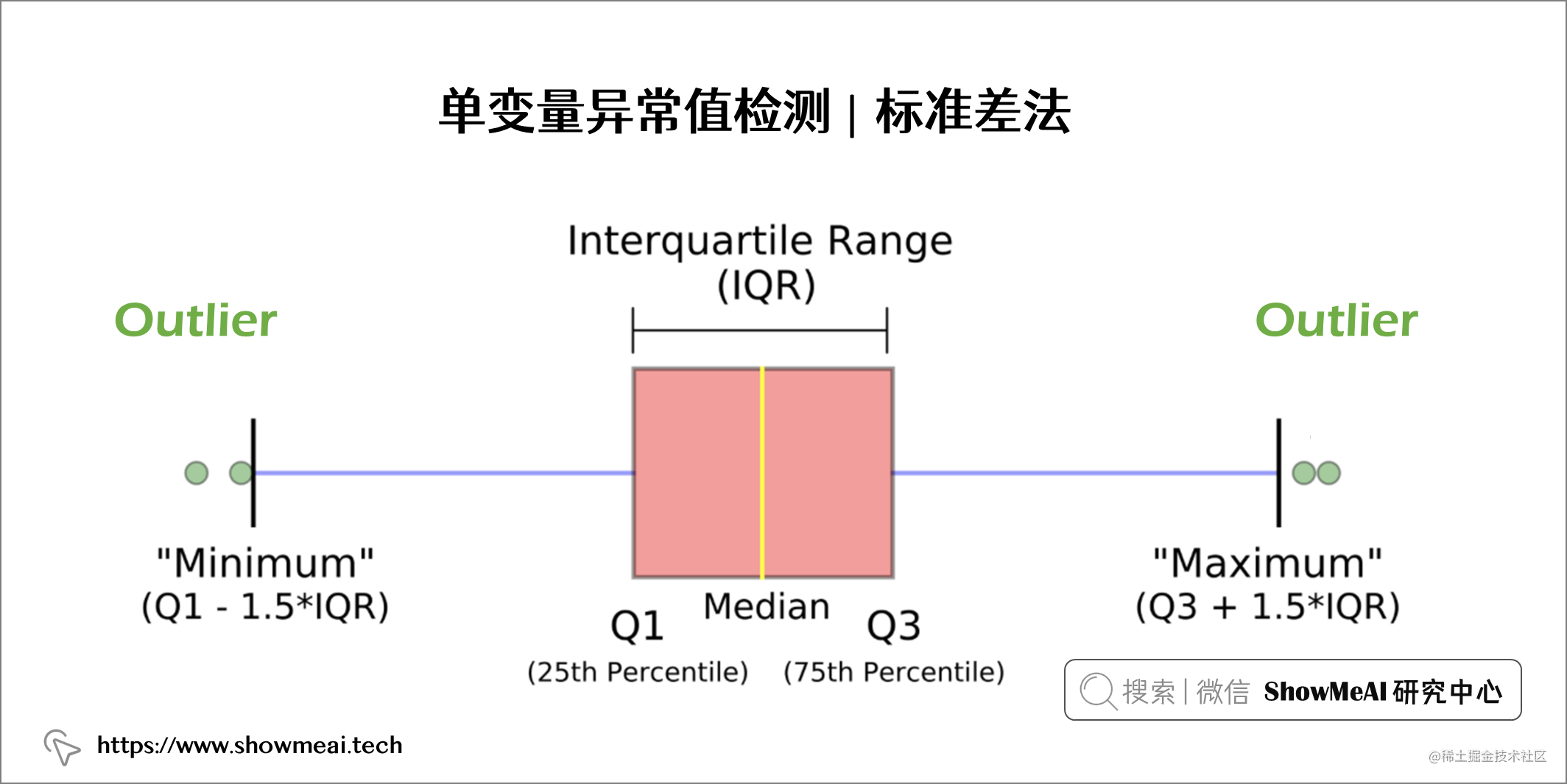``````# Find Q1, Q3, IQR and cut off value
q25, q75 = np.quantile(glass["Na"], 0.25), np.quantile(glass["Na"], 0.75)
iqr = q75 - q25
cutoff = 1.5 * iqr

# Define lower and upper boundaries
lower, upper = q25 - cutoff, q75 + cutoff

# Define new dataset by masking upper and lower boundaries
new_glass = glass[(glass["Na"] > lower) & (glass["Na"] < upper)]
Shape of original dataset: (213, 9)
Shape of dataset after removing outliers in Na column: (206, 9)

# 💡 多变量异常值检测

## 💦 孤立森林算法-Isolation Forest

📘孤立森林 是一种基于随机森林的无监督机器学习算法。我们都知道，随机森林是一种集成学习模型，它使用基模型（比如 100 个决策树）组合和集成完成最后的预估。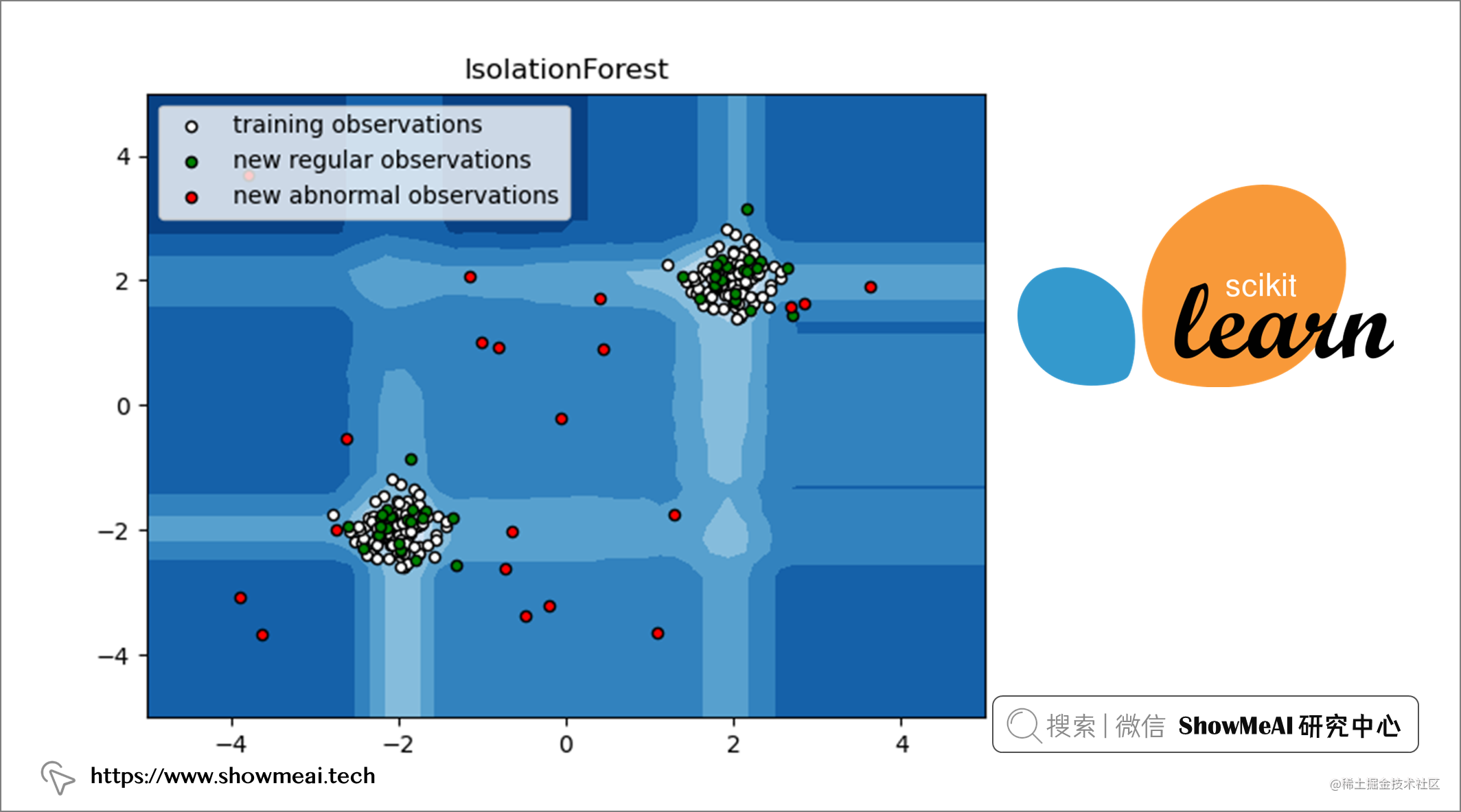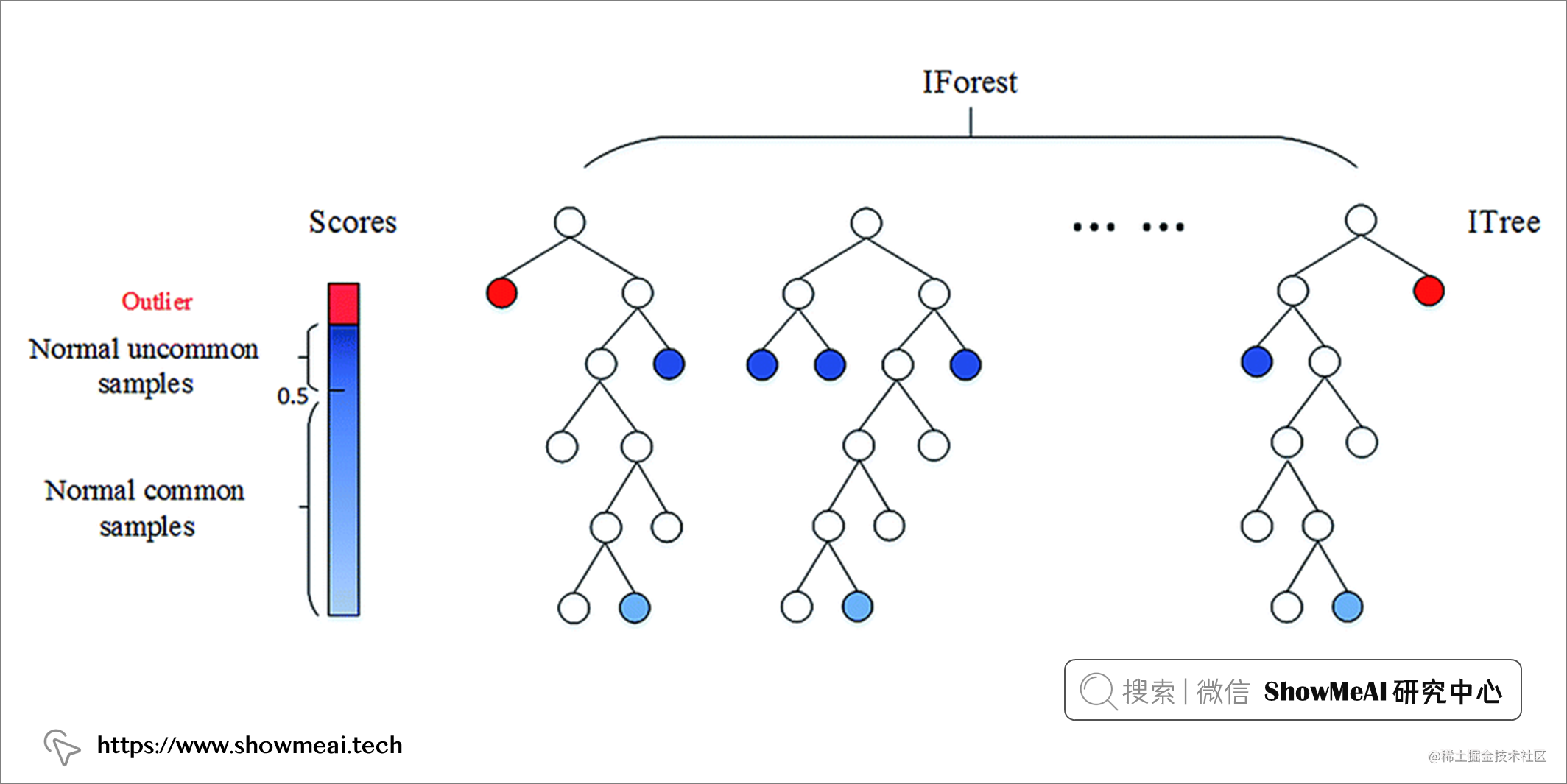``````from sklearn.ensemble import IsolationForest
IsolationForest(n_estimators=100, max_samples='auto', contamination='auto', max_features=1.0, bootstrap=False, n_jobs=None, random_state=None, verbose=0, warm_start=False)

Isolation Forest 算法有几个超参数：

• `n_estimators`：表示要集成的基模型的数量。
• `max_samples`：表示用于训练模型的样本数。
• `contamination`：用于定义数据中异常值的比例。
• `max_features`：表示采样处的用于训练的特征数。

``````from sklearn.ensemble import IsolationForest

# Initiate isolation forest
isolation = IsolationForest(n_estimators=100,
contamination='auto',
max_features=glass.shape)

# Fit and predict
isolation.fit(glass)
outliers_predicted = isolation.predict(glass)

# Address outliers in a new column
glass['outlier'] = outliers_predicted

``````1    195
-1    19
Name: outlier, dtype: int64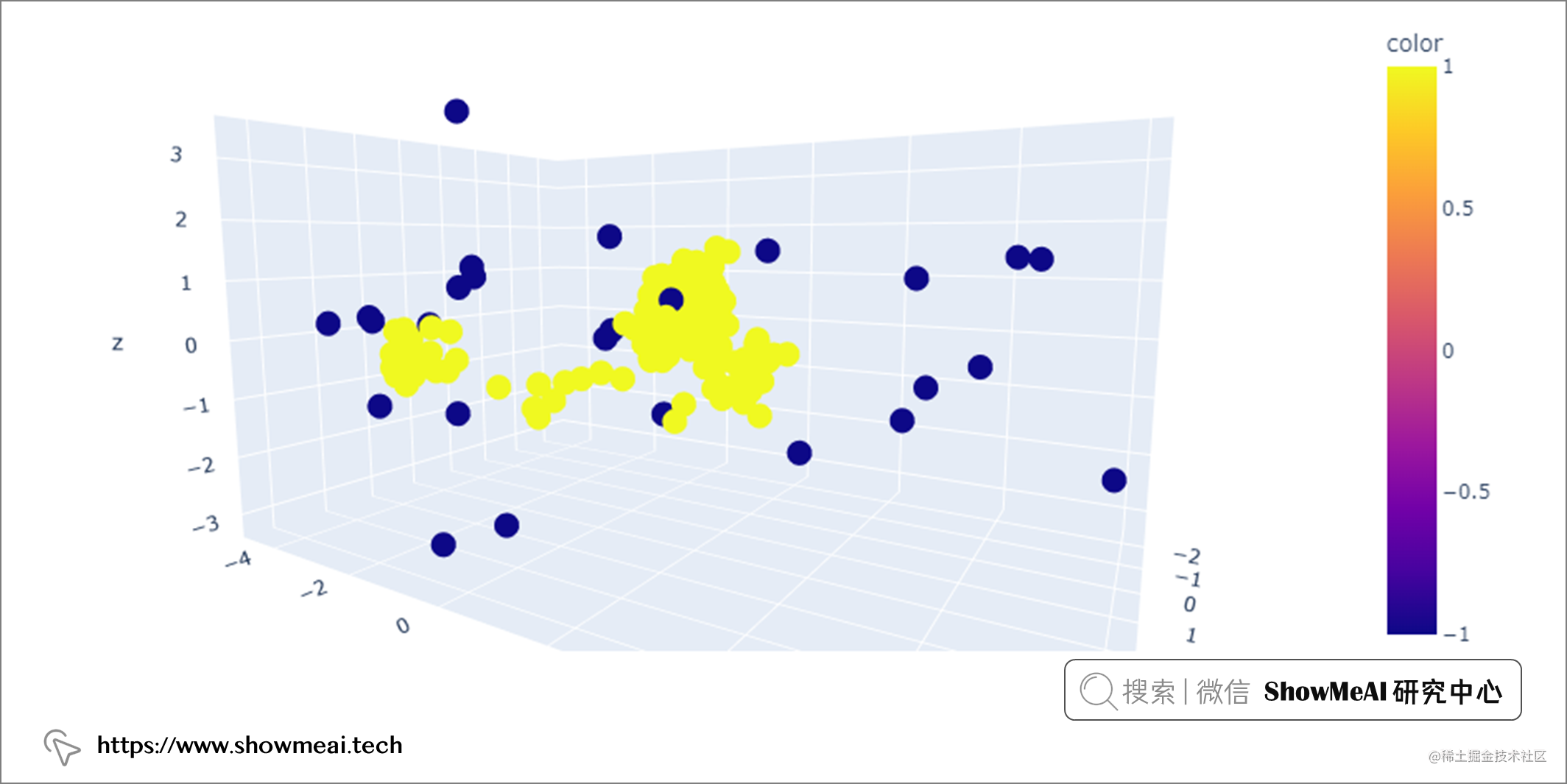## 💦 基于空间密度的聚类算法-DBSCAN

📘DBSCAN 是一种流行的聚类算法，通常用作 K-means 的替代方法。它是基于分布密度的，专注于许多数据点所在的高密度区域。它通过测量数据之间的特征空间距离（即欧氏距离）来识别哪些样本可以聚类在一起。DBSCAN 作为聚类算法最大的优势之一就是我们不需要预先定义聚类的数量。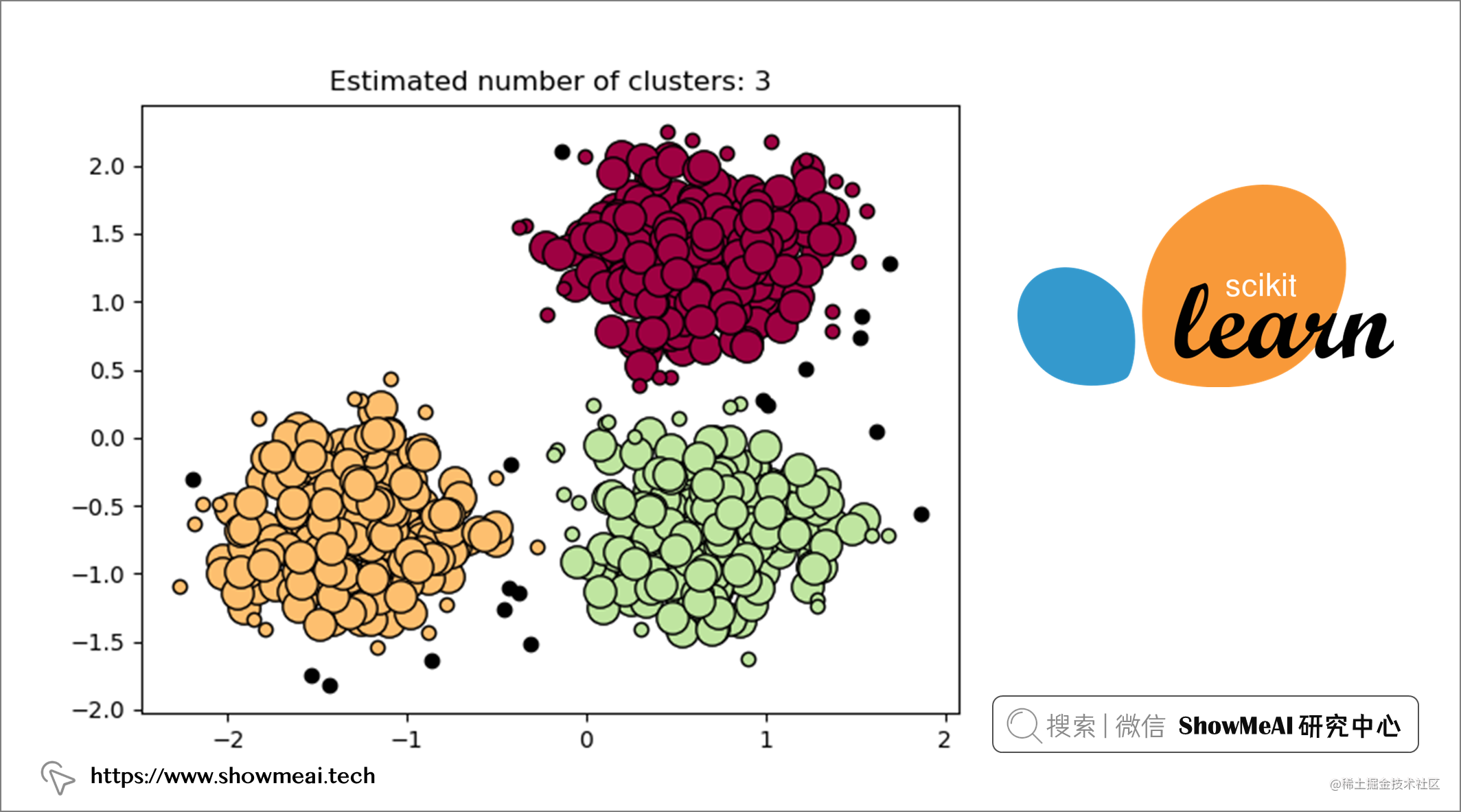``````from sklearn.cluster import DBSCAN
DBSCAN(eps=0.5, min_samples=5, metric='euclidean', metric_params=None, algorithm='auto', leaf_size=30, p=None, n_jobs=None)

DBSCAN 有几个超参数：

• `eps`（epsilon）：考虑在同一个 cluster 中的两个数据点之间的最大距离。
• `min_samples`：核心点的接近数据点的数量。
• `metric`：用于计算不同点之间的距离度量方法。

``````import numpy as np
from sklearn.cluster import DBSCAN
from sklearn.preprocessing import MinMaxScaler

# Initiate DBSCAN
dbscan = DBSCAN(eps=0.4, min_samples=10)

# Transform data
glass_x = np.array(glass).astype('float')

# Initiate scaler and scale data
scaler = MinMaxScaler()
glass_scaled = scaler.fit_transform(glass_x)

# Fit DBSCAN on scaled data
dbscan.fit(glass_scaled)

# Address outliers in a new column
glass['outlier'] = dbscan.labels_

``````import math

# Function to calculate k distance
def calculate_k_distance(X,k):

k_distance = []
for i in range(len(X)):
euclidean_dist = []
for j in range(len(X)):
euclidean_dist.append(
math.sqrt(
((X[i,0] - X[j,0]) ** 2) +
((X[i,1] - X[j,1]) ** 2)))

euclidean_dist.sort()
k_distance.append(euclidean_dist[k])

return k_distance

# Calculate and plot epsilon distance
eps_distance = calculate_k_distance(glass_scaled, 10)
px.histogram(eps_distance, labels={'value':'Epsilon distance'})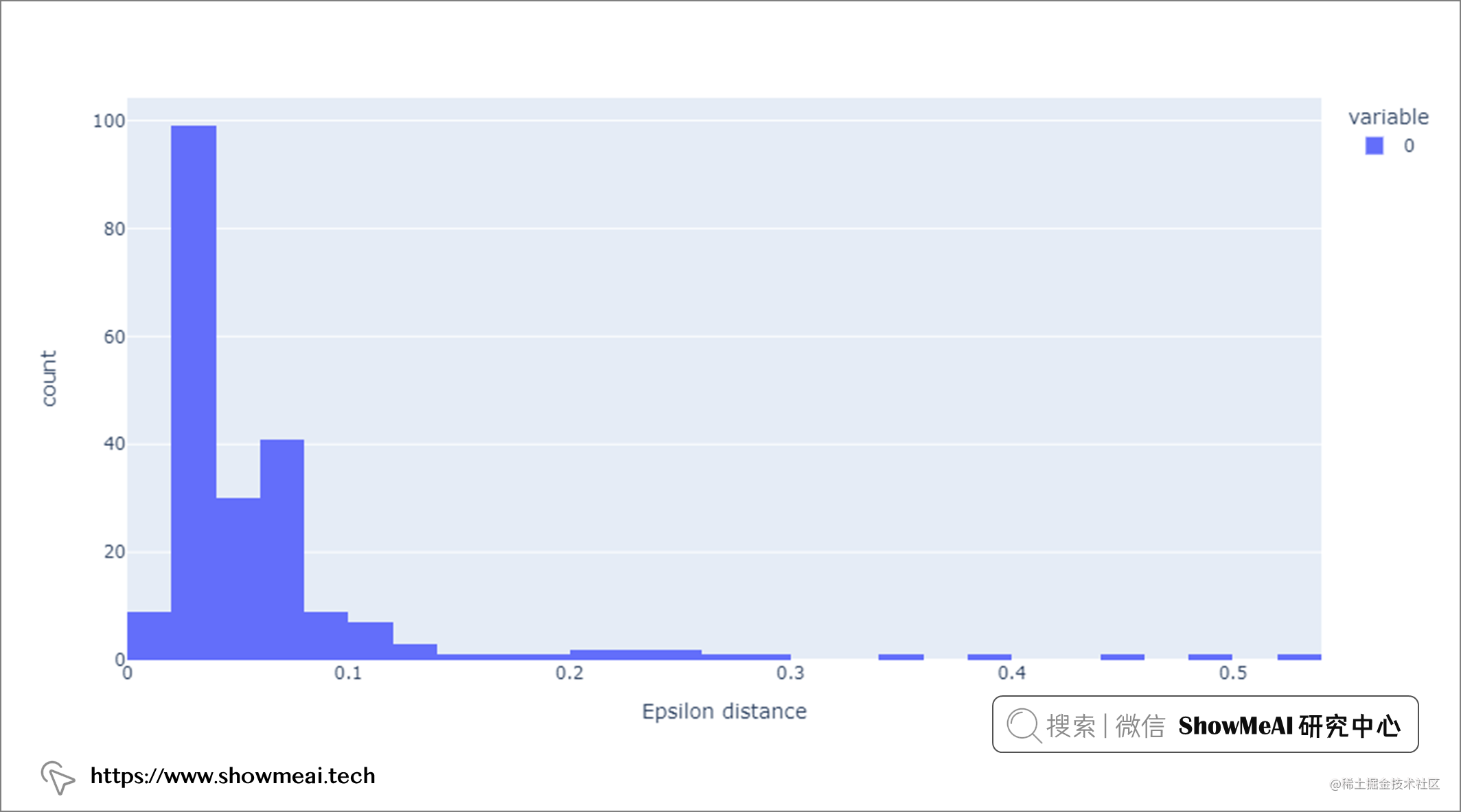``````0    192
-1    22
Name: outlier, dtype: int64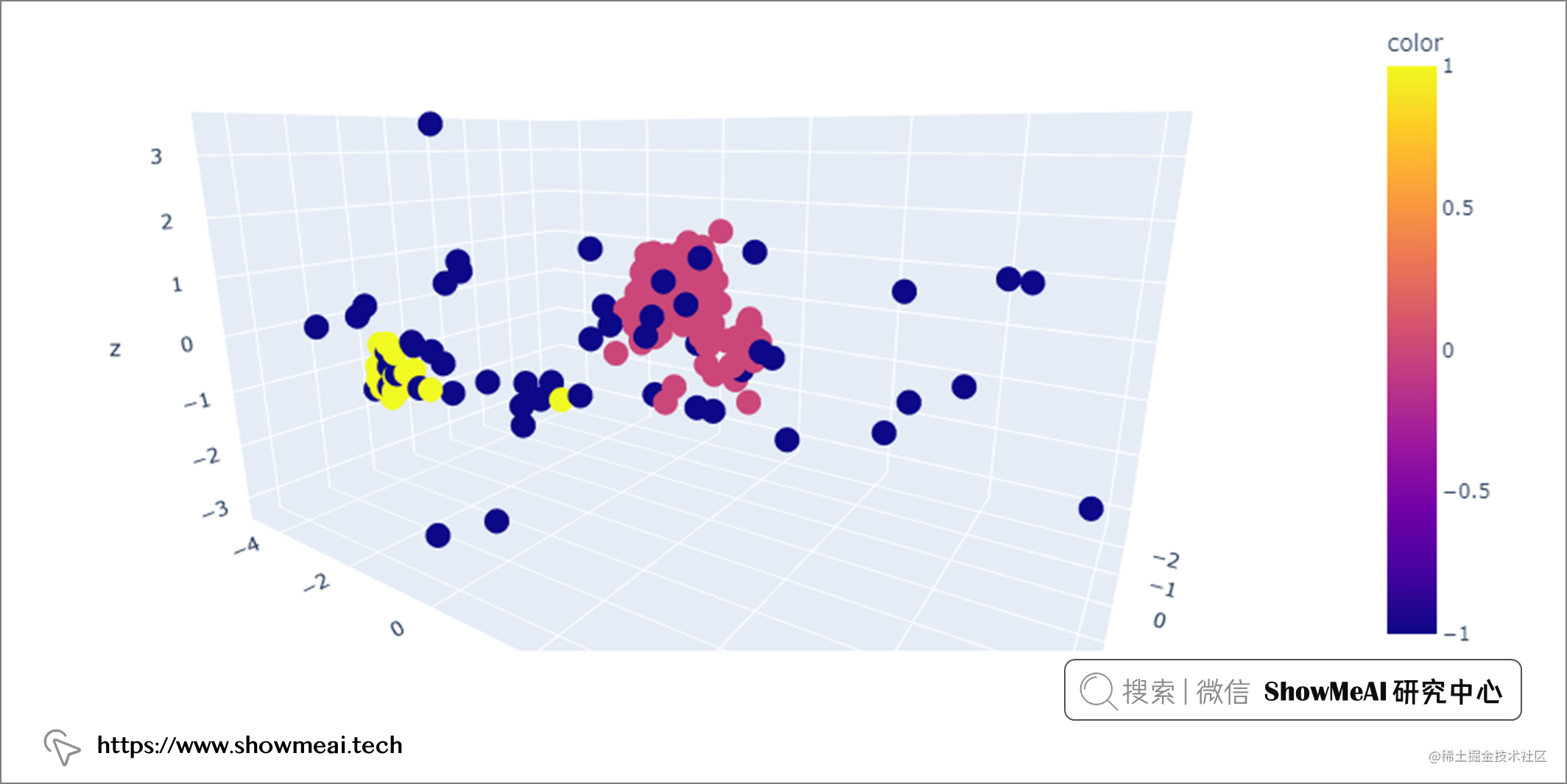## 💦 局部异常因子算法-LOF

📘LOF 是一种流行的无监督异常检测算法，它计算数据点相对于其邻居的局部密度偏差。计算完成后，密度较低的点被视为异常值。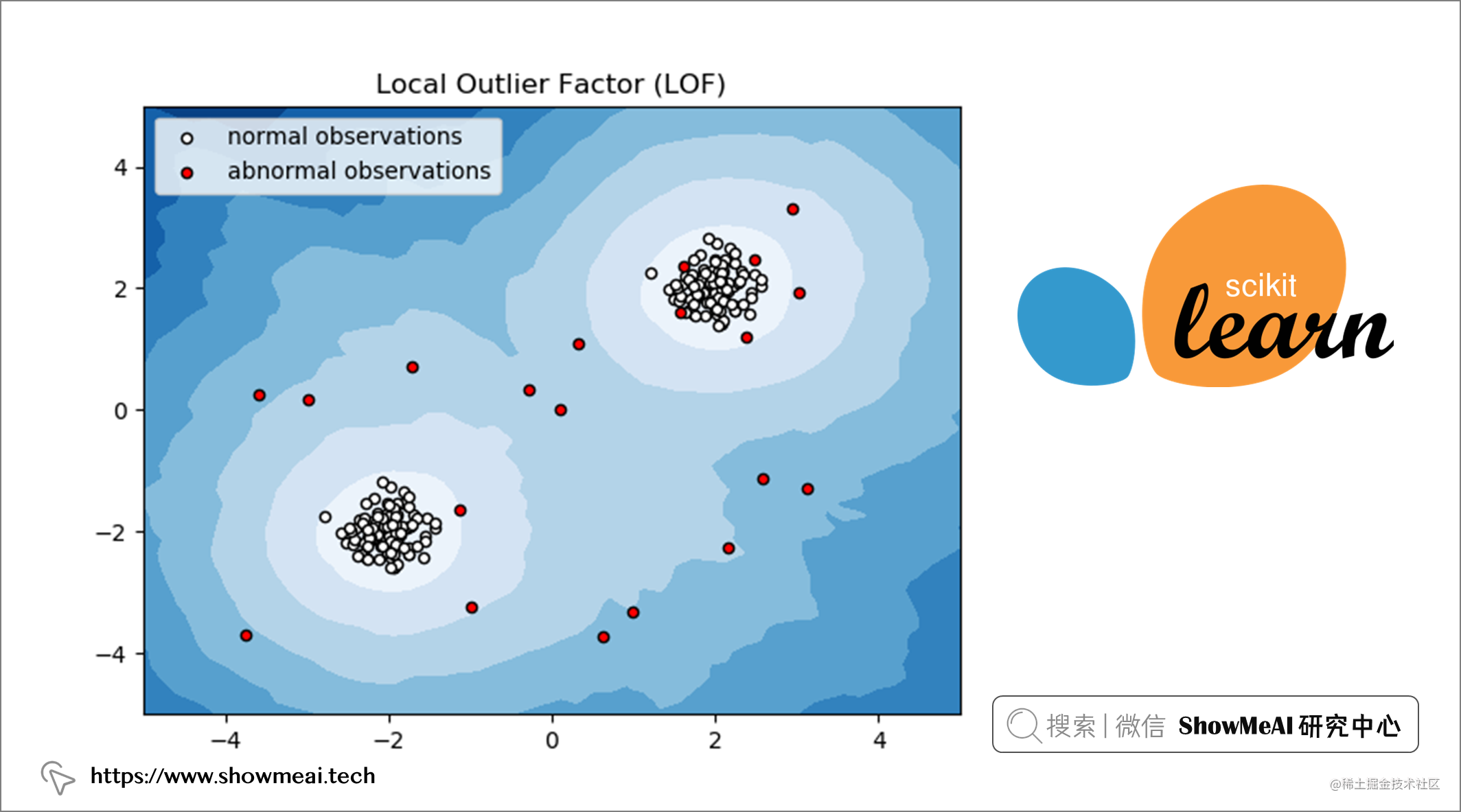``````from sklearn.neighbors import LocalOutlierFactor
LocalOutlierFactor(n_neighbors=20, algorithm='auto', leaf_size=30, metric='minkowski', p=2, metric_params=None, contamination='auto', novelty=False, n_jobs=None)

LOF 有几个超参数：

• `n_neighbors`：用于选择默认等于 20 的邻居数量。
• `contamination`：用于定义离群值比例。
``````from sklearn.neighbors import LocalOutlierFactor

# Initiate LOF
lof = LocalOutlierFactor(n_neighbors=20, contamination='auto')

# Transform data
glass_x = np.array(glass).astype('float')

# Initiate scaler and scale data
scaler = MinMaxScaler()
glass_scaled = scaler.fit_transform(glass_x)

# Fit and predict on scaled data
clf = LocalOutlierFactor()
outliers_predicted = clf.fit_predict(glass)

# Address outliers in a new column
glass['outlier'] = outliers_predicted

LOF建模完成后，通过调用`glass['outlier'].value_counts()`我们可以看到有 34 条记录被标记为`-1`（异常值），其余 180 条记录被标记为`1`（正常值）。

``````1    180
-1    34
Name: outlier, dtype: int64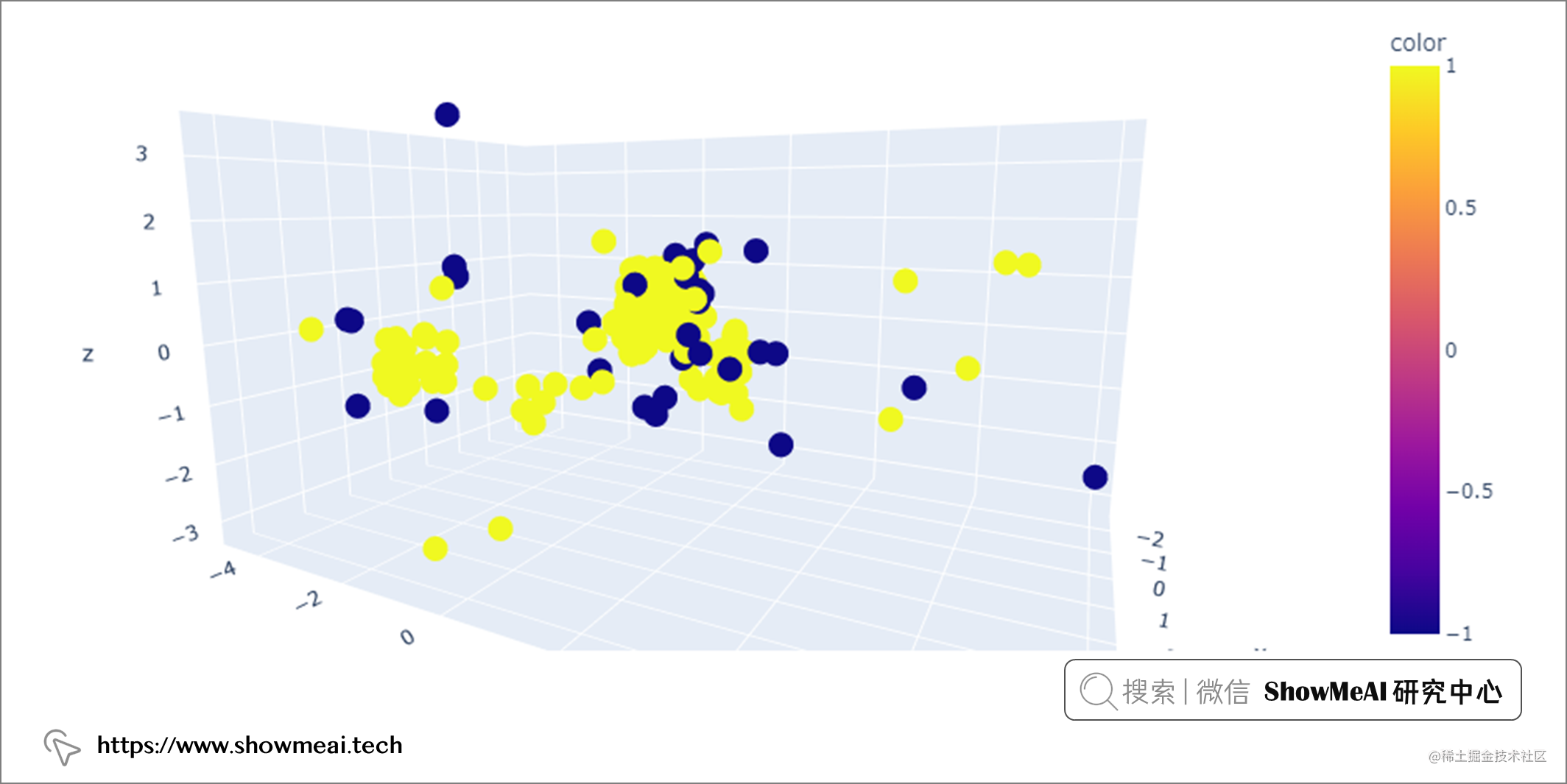# 推荐阅读